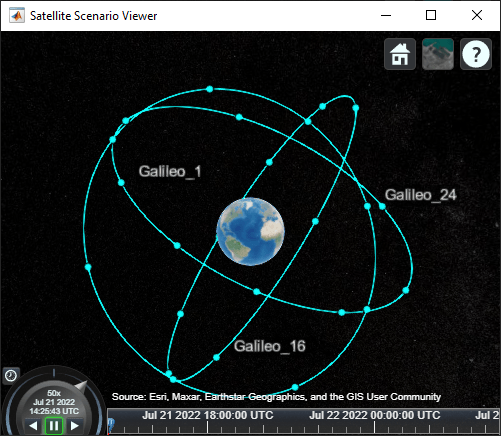# walkerDelta

Create Walker-Delta constellation in satellite scenario

## Syntax

``sat = walkerDelta(scenario,radius,inclination,totalSatellites,geometryPlanes,phasing)``
``sat = walkerDelta(__,Name=Value)``

## Description

example

````sat = walkerDelta(scenario,radius,inclination,totalSatellites,geometryPlanes,phasing)` creates an array of satellites, `sat`, inside the satellite scenario `scenario` using specified geometric properties such as radius, `radius`, inclination, `inclination`, total number of satellites, `totalSatellites`, number of geometry planes, `geometryPlanes`, and phasing between satellites, `phasing`. For more information on Walker-Delta constellations, see Algorithms.`sat = walkerDelta(__,Name=Value)` creates an array of satellites using one or more optional `Name=Value` arguments. Use this option with any of the input argument combinations in the previous syntax.```

## Examples

collapse all

Model a Galileo constellation as a Walker-Delta constellation that contains 24 satellites in three planes inclined at 56 degrees (56:24/3/1) in a 29599.8 km orbit. Provide an initial argument of latitude offset of 15 degrees.

Create a default satellite scenario object.

`sc = satelliteScenario;`

Create Walker-Delta constellation that contains 24 satellites in three planes inclined at 56 degrees (56:24/3/1) in a 29599.8 kilometer orbit.

```sat = walkerDelta(sc, 29599.8e3, 56, 24, 3, 1, ... ArgumentOfLatitude=15, Name="Galileo");```

Visualize the scenario using the Satellite Scenario Viewer.

`satelliteScenarioViewer(sc);`## Input Arguments

collapse all

Satellite scenario, specified as a `satelliteScenario` object.

Orbital radius, specified as a scalar, in meters.

Data Types: `double`

Inclination, specified as a scalar, in degrees.

Data Types: `double`

Total number of satellites, specified as a scalar positive integer.

Data Types: `double`

Number of equally spaced geometry planes, specified as a scalar positive integer.

Data Types: `double`

Phasing between satellites in adjacent planes, specified as a scalar integer greater than or equal to 0 and less than `geometryPlanes`. The change in true anomaly for equivalent satellites in neighboring planes is calculated as:

`(phasing*360/totalSatellites).`

Data Types: `double`

### Name-Value Arguments

Specify optional pairs of arguments as `Name1=Value1,...,NameN=ValueN`, where `Name` is the argument name and `Value` is the corresponding value. Name-value arguments must appear after other arguments, but the order of the pairs does not matter.

Example: `sat = walkerDelta(sc,29599.8e3,56, 24,3,1,Name="Galileo")` creates an array of satellites named Galileo.

Right ascension of ascending node, specified as a scalar value from 0 to 360. RAAN is the angle in the equatorial plane from the x-axis to the location of the ascending node, point at which the satellite crosses the equator from south to north, in degrees. The function uses this value as the starting point for distribution of the satellites along the equator.

Data Types: `double`

Argument of latitude, specified as a scalar value from 0 to 360. `ArgumentOfLatitude` is the angle between the ascending node and the body. The function uses this value as the starting point for distribution of satellites along the first orbital track.

Data Types: `double`

Constellation name, specified as a scalar.

Individual satellite names within the constellation use the constellation name appended with increasing whole numbers starting with 1, for example, `Name_1`, `Name_2`, and so forth.

Data Types: `char` | `string`

Name of orbit propagator used for propagating satellite position and velocity, specified as:

• `"two-body-keplerian"` — Two-Body-Keplerian orbit propagator based on the relative two-body model that assumes a spherical gravity field for the Earth and neglects third body effects and other environmental perturbations. It is considered the least accurate.

• `"sgp4"` — Simplified General Perturbations-4 orbit propagator.

• `"sdp4"` — Simplified Deep-Space Perturbations-4 orbit propagator.

Data Types: `string` | `char`

## Output Arguments

collapse all

Satellite in scenario, returned as an array of `Satellite` objects.

## Algorithms

Walker-Delta constellations are a common solution for maximizing geometric coverage over Earth while minimizing the number of satellites required to perform the mission. Walker-Delta constellation patterns use the notation:

`I:T/P/F.`
where:

• `I` — Orbital inclination

• `T` — Total number of satellites, which must be divisible by `F`

• `P` — Number of equally spaced geometric planes

• `F` — Phasing between satellites in adjacent planes

To define the radial height of the circular orbit (with respect to the Earth center), the function also requires a radius `r`.

• The ascending nodes of the orbital planes of a Walker-Delta constellation are uniformly distributed at intervals of 360/`P` deg around the equator.

• The number of satellites per plane, `satellitesPerPlane`, is defined as

`satellitesPerPlane=T/P.`

The satellites in each orbital plane are distributed at intervals of 360/`satellitesPerPlane` deg. `F` represents the interplane phasing, the number of empty slots between the first satellites in each orbital plane.

## Version History

Introduced in R2022a